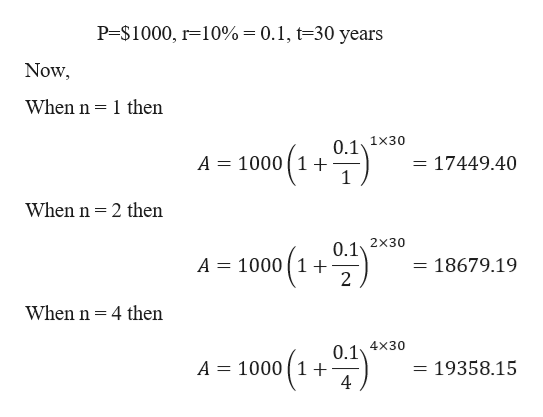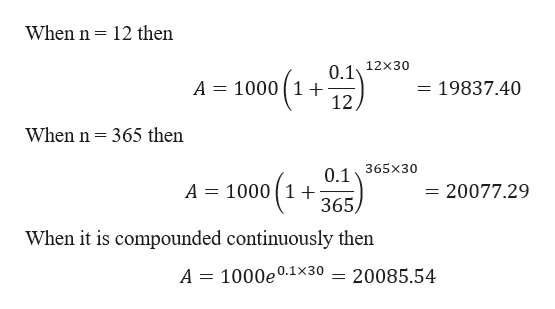n12412365continuousA      Complete the table to find the balance A for P dollars invested at rate r for t years,compounded n times a year. Round your answers to the nearest centP=\$1000, r=10% , t=30 years

Question
 n 1 2 4 12 365 continuous A

Complete the table to find the balance A for P dollars invested at rate r for t years,compounded n times a year. Round your answers to the nearest cent

P=\$1000, r=10% , t=30 years

Step 1

Given,help_outlineImage TranscriptioncloseP=\$1000, r 10%= 0.1, t=30 years Now When n 1 then 0 11x30 A 1000 1+ 1 17449.40 When n 2 then 0 12x30 A 1000 1+ 2 18679.19 When n 4 then 0.14x30 A 1000 1+ = 19358.15 11 fullscreen
Step 2help_outlineImage TranscriptioncloseWhen n 12 then 0 1 12x30 A 1000 1 12, 19837.40 When n 365 then 0.1365x30 A = 1000 1 + 365 20077.29 When it is compounded continuously then 20085.54 A = 1000e0.1x30 fullscreen

Want to see the full answer?

See Solution

Want to see this answer and more?

Our solutions are written by experts, many with advanced degrees, and available 24/7

See Solution
Tagged in

Other